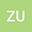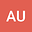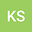Computation of Semi-Analytical Solutions to Fuzzy Non-linear Integral Equations
•••• +1
• Zia Ullah,
• Aman Ullah,
• Kamal Shah,
• D. Baleanu
Zia Ullah
University of Malakand
Author ProfileAman Ullah
University of Malakand
Author ProfileKamal Shah
University of Malakand
Author ProfileD. Baleanu
Cankaya Universitesi
Author Profile## Abstract

In this article, we use a fuzzy number in its parametric form and transform a non-linear fuzzy integral equations to its parametric form of the second kind as in the crisp case. The main focus is to solve the fuzzy non-linear integral equations for semi analytical solutions. The suggested treatment are presented for the solution of respective fuzzy non-linear integral equations including fuzzy non-linear Fredholm integral equation, fuzzy non-linear Volterra integral equations and fuzzy non-linear singular integral equation of Able's type kernel via an hybrid method of integral transform and decomposition technique. The proposed method is illustrated in details by solving few examples.